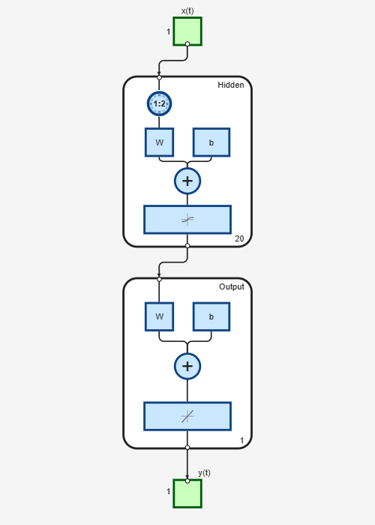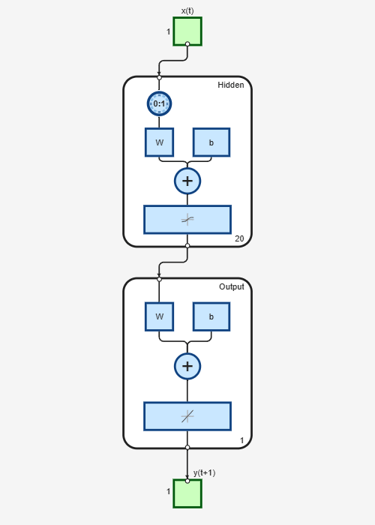Add delay to neural network response

Description

net = adddelay(net,n) takes these arguments,

 net Neural network n Number of delays

and returns the network with input delay connections increased, and output feedback delays decreased, by the specified number of delays n. The result is a network that behaves identically, except that outputs are produced n timesteps later.

If the number of delays n is not specified, a default of one delay is used.

Examples

Time Delay Network

This example creates, trains, and simulates a time delay network in its original form, on an input time series X and target series T. Then the delay is removed and later added back. The first and third outputs will be identical, while the second result will include a new prediction for the following step.

[X,T] = simpleseries_dataset;
net1 = timedelaynet(1:2,20);
[Xs,Xi,Ai,Ts] = preparets(net1,X,T);
net1 = train(net1,Xs,Ts,Xi);
y1 = net1(Xs,Xi);
view(net1)net2 = removedelay(net1);
[Xs,Xi,Ai,Ts] = preparets(net2,X,T);
y2 = net2(Xs,Xi);
view(net2)